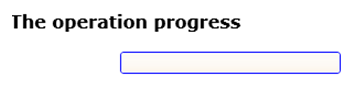Post

# Implementing a Progress Bar in Silverlight 2

• 25k
• 0
• 1

The ProgressBar represents a perfect tool to illustrate the task progress to the user. It could be configured according to two styles, namely the marquee style and the normal or the classic style. The first one is used when the task achievement time is undetermined. At the contrast, the classic style within which the bar is filled based on a given value.  The ProgressBar could be configured using the IsIndeterminate property. The value itself could be set through the Value property and it is limited by using the Minimum and the Maximum properties. The Minimum value couldn't be less than 0 and the Maximum one couldn't be more than 100. The bellow example illustrates how to use the ProgressBar according to the classic style. First the following XAML should be implemented.

<StackPanel x:Name="LayoutRoot"

Orientation="Vertical"

Background="White" >

<Line Height="30"/>

<TextBlock

FontFamily="Time New Romain"

FontSize="16"

FontWeight="Bold">

The operation progress

</TextBlock>

<ProgressBar x:Name="myBar"

Foreground="Cyan"

Background="AntiqueWhite"

BorderBrush="Blue"

Maximum="100"

Width="200"

Height="20"

Margin="15"/>

<TextBlock x:Name="myTextBloc"

Margin="15,15"></TextBlock>

</StackPanel>

The interface will be as in the Figure 1Figure 1

Then the following code behind has to be implemented

public partial class Page : UserControl

{

ProgressBar oBar;

Storyboard oTimer;

TextBlock Result;

public Page()

{

InitializeComponent();

InitializeBehaviour();

}

public void InitializeBehaviour()

{

oBar = this.FindName("myBar") as ProgressBar;

oTimer = new Storyboard();

Result = this.FindName("myTextBloc") as TextBlock;

oTimer.Duration = new Duration(new TimeSpan(10));

oTimer.Completed+=new EventHandler(oTimer_Completed);

oTimer.Begin();

}

Random x = new Random();

public void oTimer_Completed(object sender, EventArgs e)

{

int period = x.Next(1, 5)*100;

if (oBar.Value < oBar.Maximum)

{

oBar.Value += 1;

oTimer.Begin();

}

if (oBar.Value >= oBar.Maximum)

Result.Text = "Operation accomplished";

}

}

If the application is fired up, the progress bar will be filled increasingly until the end, and then and information is displayed to the user in order to inform him that the given task is finished. Another important member that shouldn't be missed within this context, it is the ValueChanged event.Figure 2

It could be used for example to illustrate the progress percentage during the operation achievement. In this case, the code behind will be.

public void InitializeBehaviour()

{

oBar = this.FindName("myBar") as ProgressBar;

oTimer = new Storyboard();

Result = this.FindName("myTextBloc") as TextBlock;

oTimer.Duration = new Duration(new TimeSpan(10));

oTimer.Completed+=new EventHandler(oTimer_Completed);

oTimer.Begin();

oBar.ValueChanged+=new RoutedPropertyChangedEventHandler<double>(oBar_ValueChanged);

}

private void oBar_ValueChanged(object sender, RoutedPropertyChangedEventArgs<double> e)

{

Result.Text = "Operation progress:  " + e.NewValue.ToString() + " %";

}

Random x = new Random();

public void oTimer_Completed(object sender, EventArgs e)

{

int period = x.Next(1, 5)*100;

if (oBar.Value < oBar.Maximum)

{

oBar.Value += 1;

oTimer.Begin();

}

if (oBar.Value >= oBar.Maximum)

Result.Text = "Operation accomplished";

}

And then the result will beFigure 3

That's it.

Good Dotneting!!!

Recommended Free Ebook
Similar Articles